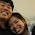## Saturday, August 27, 2011

### Do the Primes go to Infinity and Beyond?!!

We've been doing a lot of work with patterns, or more formally called sequences or infinite series. Infinite series are a little different though, as they are guaranteed to go on forever without stopping. For instance, the sequence with formula n^2, or 1, 4, 9, 16..., is an infinite series. However, one like √-n + 3 is not infinite, as it will go √2, 1, 0, and then will be hitting complex numbers, which are not valid for these sequences.

Most patterns, it is pretty obvious, and would never be on a test, or even a valid question for a teacher to ask. However, what about the prime numbers. If you don't know, primes are numbers with only two factors, one and itself. So, 5 is prime, because its factors are 1 and 5. However, 6 is not prime, because it has more than two factors, namely 1, 2, 3, and 6. That means that 6 is composite, which is having three or more factors. Numbers such as one are known as universal, as they have only one factor.

Anyways, are prime numbers infinite? This is definitely a valid question, and answerable too! Can you create a list of all prime numbers on it? How about you try to. I'll bet you can't.

Say someone pops up and says,"I have made a list with all of the prime numbers that exist on it." The list would be much longer if someone did say that, but I made a small list below:

2
3
5
7
11

Okay. Let's multiply all of these numbers you've found together. 2 x 3 x 5 x 7 x 11 = 2310. Great. According to you, this is the product of all of the prime numbers out there. Try adding one. Now, we have 2311, which is a multiple of no prime numbers. Since every number is composed of primes, or is prime, this is not possible. That means the number is either prime, as 2311 happens to be, or could be a multiple of another prime that is not present on the list.

Is that all of the primes? No! We can do that process forever, and always find a prime that is missing. This is not a formula to generate prime numbers, as the first ten primes multiplied together is 6,469,693,230 which if you add one gives you 6,469,693,231, which you have no clue if it is prime or not! You guys can figure that one out. However, it is a cool proof that answers a question that definitely gets you thinking!

#### 1 comment:

1.